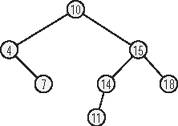### 12.5. Characteristics

A binary tree consists of a number of nodes that contain the data to be stored (or pointers to the data), and the following structural characteristics :

• Each node has up to two direct child nodes.

• There is exactly one node, called the root of the tree, that has no parent node. All other nodes have exactly one parent.

• Nodes in a binary tree are placed according to this rule: the value of a node is greater than or equal to the values of any descendant in its left branch, and less than the value of any descendant in its right branch.

Figure 12-1 illustrates the structure of a binary tree.

##### Figure 12-1. A binary treeA leaf is a node that has no children. Each node of the tree is also considered as the root of a subtree, which consists of the node and all its descendants.

An important property of a binary tree is its height. The height is the length of the longest path from the root to any leaf. A path is a succession of linked nodes that form the connection between a given pair of nodes. The length of a path is the number of nodes in the path, not counting the first node. It follows from these definitions that a tree consisting only of its root node has a height of 0, and the height of the tree in Figure 12-1 is 3.